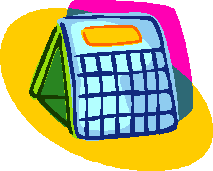#### You may also like### Prompt Cards

These two group activities use mathematical reasoning - one is numerical, one geometric.### Consecutive Numbers

An investigation involving adding and subtracting sets of consecutive numbers. Lots to find out, lots to explore.### Exploring Wild & Wonderful Number Patterns

EWWNP means Exploring Wild and Wonderful Number Patterns Created by Yourself! Investigate what happens if we create number patterns using some simple rules.

# Calendar Calculations

##### Age 7 to 11Challenge LevelFind the sum of all the dates in a week (you could use the dates of this week).

Write down the total.

Multiply the number of the first day in the week by $7$.

Add $21$ to the product.

Write down the total.

What do you notice about the two results?

Can you explain what accounts for the second answer?

Choose two consecutive weeks on the calendar and find the sum of the dates.

Multiply the number of the first day in the first week by $14$.

Add $91$ to the product.

How does this compare with using just one week?

Why do you multiply by $14$ and why add $91$?